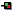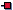## Enumerations

J2SE 5.0 introduced the concept of enumerations. Enumerations at their simplest are lists of constants represented by an identifier. The `enum` keyword has been added to the language to allow us to create enumeration lists. This operates in a similar fashion to the C/C++ equivalent. Consider this example:

```  public static final int SUNDAY = 0;
public static final int MONDAY = 1;
public static final int TUESDAY = 2;
...
```

that is one way that we could define a set of states/constants. However, this approach has a few problems. The values are not type safe, so, if a day of the week is required as a parameter to a method, it would be passed as an int. This would allow us to pass the int value `25`, or indeed we could pass `MONDAY+TUESDAY`, which clearly makes no sense. Also, if you print out the value, you will get an uninformative `0` to represent Sunday, or Monday? Well, Sunday in this case - but you have to remember that. A better way (and less verbose way) to do this is:

```  private enum Day { SUNDAY, MONDAY, TUESDAY, WEDNESDAY,
THURSDAY, FRIDAY, SATURDAY };
```

Here is a full example of the use of enumerations. This example is available in `EnumerationExample.java`:

``` 1
2 public class EnumerationExample
3 {
4 	// just for a sample - as per previous example
5 	private enum Day { SUNDAY, MONDAY, TUESDAY, WEDNESDAY,
6 	   THURSDAY, FRIDAY, SATURDAY };
7
8 	// now for this example
9 	private enum Drive { TURN_LEFT, TURN_RIGHT, STOP };
10
11 	public static void main(String args[])
12 	{
13 	  System.out.println("Enumeration Example:");
14 	  Drive driveInstruction = Drive.STOP;
15
16 	  switch(driveInstruction)
17 	  {
18 	    case TURN_LEFT:
19 	      System.out.println("Turning left...");
20 	      break;
21 	    case TURN_RIGHT:
22 	      System.out.println("Turning right...");
23 	      break;
24 	    case STOP:
25 	      System.out.println("Stopping...");
26 	      break;
27 	    default:
28 	      System.out.println("Invalid Instruction");
29 	      break;
30 	  }
31 	  System.out.println("End of enumeration example.");
32 	}
33 }
34 ```

This program demonstrates how we can create an enumeration; in this case we create an enumeration for `Drive`, which consists of three "states" `TURN_LEFT``TURN_RIGHT` and `STOP`. Once we have this enumeration we are able to use it in a type safe way. For example, there is no possibility of each state variable having the same underlying value; they are completely independent of each other. We can also create type safe variables of the `Drive` type. In this example, `Drive driveInstruction` can only be assigned a value of `Drive.TURN_LEFT``Drive.TURN_RIGHT` and `Drive.STOP`. The same would be true if this was a parameter of a method to be received, where a value such as `324` could be provided, which would be invalid but not detected at compile time.

However, enumerations in Java (since J2SE 5.0) are more than just lists of values. In fact, the keyword `enum` allows us to create a special type of class. This allows us to add data and behaviour to an `enum`type, just as you would to a class.

Here is an example of a enum type`ConversionFactor`:

``` 1
2 enum ConversionFactor
3 {
4   MILESTOKILOMETRES (1.609344),
5   KILOMETRESTOMILES (0.621371192),
6   KILOGRAMSTOPOUNDS (2.20462262),
7   POUNDSTOKILOGRAMS (0.45359237);
8
9   private final double factor;
10
11   ConversionFactor(double factor)
12   {
13     this.factor = factor;
14   }
15
16   public double getFactor()   { return factor; }
17
18   public double convert(double value)
19   {
20     return this.factor * value;
21   }
22 }
23 ```

Here the `enum` type defines several conversions, e.g. "What is 5 miles in kilometers?" to convert between units of distance and weight. In this example the `enum` type has a constructor`ConversionFactor()` that accepts a double value. In this example each `enum` constant is actually an object of type`ConversionFactor` that is created by an automatic individual call to the `enum` type constructor. It is possible for the `enum` constants to have several different values in the form: `CONSTANT ( value1, value2, value3),` of mixed types. These values would then be passed in the correct order to the constructor of the enum type class. In this example, I have written a method`getFactor()` that returns the current conversion factor, and a method`convert()` that allows us to convert adouble variable by the chosen conversion factor. Here is an example of a`main()` method that uses the `enum` type above:

``` 1
2 public class EnumerationExample2
3 {
4   public static void main(String args[])
5   {
6     System.out.println("Enumeration Example:");
7     double valueToConvert = Double.parseDouble(args);
8     for (ConversionFactor c : ConversionFactor.values())
9     {
10         System.out.printf("The Value from %s is %f%n",
11            c, c.convert(valueToConvert));
12     }
13     System.out.println("End of enumeration example.");
14   }
15 }
16 ```

In this example we take the first command line argument and convert it according to all of the conversion factors that are defined in the `enum` type, i.e. Miles->Kilometers, Kilometers->Miles, Kilograms->Pounds(UK) and Pounds(UK)->Kilograms. We read in the value from the command line and then use a for-each loop (as discussed before) to iterate over all of the values in the`ConversionFactor` enum type. Each `enum` type has a static`values()` method that returns an array containing all of the values of the `enum` type in the order that they are declared. This is commonly used in combination with the for-each loop to iterate over the values of an enumerated type.

The full source code for this example is available in: `EnumerationExample2.java`. When executed with the command java EnumerationExample2 100 it will result in the output:

```Enumeration Example:
The Value from MILESTOKILOMETRES is 160.934400
The Value from KILOMETRESTOMILES is 62.137119
The Value from KILOGRAMSTOPOUNDS is 220.462262
The Value from POUNDSTOKILOGRAMS is 45.359237
End of enumeration example.
```

The `enum` type is very useful for binding methods and behaviour to a list of constants, thus associating certain functionality with these constants. We could use this structure for the "Periodic Table of Elements" and add methods to carry out required calculations on the values obtained from this table of elements. Other examples would include the planets, units of measurement, material properties, building regulations, music notes, letters of the alphabet - basically any set of constants about which we need to perform calculations and/or conversions.

In Java we can even define our `enum` constants to provide a different behaviour for a particular method. In this example I have provided four mathematical operations and a method called`eval()` that performs the operation in question. This example could be used instead of numerous `switch()` calls. It would be very useful if you were writing some form of application where rules had to be loaded from a file and executed on your own "state machine". Here is such an example:

``` 1
2 import static java.lang.Math.*;
3
4 enum Operation
5 {
6 	SQRT { double eval(double x) { return sqrt(x); } },
7 	LOG  { double eval(double x) { return log(x);  } },
8 	CEIL { double eval(double x) { return ceil(x); } },
9 	SIN  { double eval(double x) { return sin(x);  } };
10
11 	abstract double eval(double x);
12 }
13
14 public class EnumerationExample3
15 {
16   public static void main(String args[])
17   {
18     double x = Double.parseDouble(args);
19     for (Operation op : Operation.values())
20       System.out.printf("%s %f = %f%n", op, x, op.eval(x));
21   }
22 }
23
24 ```

In this example the operation is performed on the argument and the operation itself is displayed in the output, as demonstrated below. I have used `import static` here as a reminder example that we can import a class in a static way, thus removing the need for calls to `Math.sqrt()` etc. The full source code for this example is available in: `EnumerationExample3.java`. When executed with the command java EnumerationExample2 5.554 it will result in the output:

```C:\>java EnumerationExample3 5.554
SQRT 5.554000 = 2.356693
LOG 5.554000 = 1.714518
CEIL 5.554000 = 6.000000
SIN 5.554000 = -0.666262```